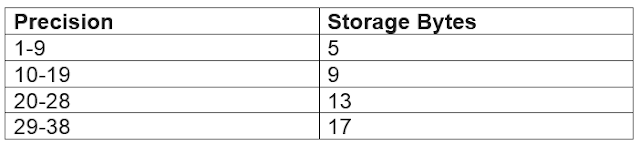SQL Server: Data Types - Part 1 - H Y R Tutorials
Question: Do we have data types in SQL server?

Answer: Yes, we have data types in SQL server.

Now will see what the data types are and how to use these data types.

Data Type:

A data type is an attribute that specifies what type of data column can hold in it like integer data or character data or string data or date and time data etc...

SQL server supplies a set of system data types that defined all the types of data that can be stored within SQL server data types in SQL server are organized into the following categories:

Exact numeric

Approximate numeric

Date and time

Character strings

Unicode character strings

Binary strings

Other data types

Integer Types:

These are the Exact number of data types that stores integer dataMonetary or Currency Types:

Data types that represent monetary or currency valuesBit (Boolean Type):

It is an integer type data type that can store the values like 10 or null

Note: the string values true and false or converted to big palace true is converted to 1 and false is converted to zero converting to bit promotes any non zero value to 1

Decimal Types:

Numeric data types that have fixed Precision and scale

Syntax: decimal[(p[,s])] and numeric[(p[,s])]

P(precision):

The maximum total number of decimal digits that can be stored post to the left-hand side and to the right-hand side of the decimal point the precision must be a value from 1 through the maximum position of 38 the default position is 18

S(scale)

The maximum number of decimal digits that can be stored to the right of the decimal point scale must be a value from zero to pay scale can be specified only if precision is specified the default scale is gyro maximum storage size is variable based on the precision as followingFloat[(n)] and Real:

Approximate number of data types for use with floating point numeric data

floating point is approximate therefore not all the values in the data type can be represented exactly where n is the number of bits that are used to store the mantissa of the float number in scientific notation and therefore dictates the precision and storage size if you any specified it must be a value between 1 and 53 the default value of n is 53In my next article, I will explain about remaining data types.